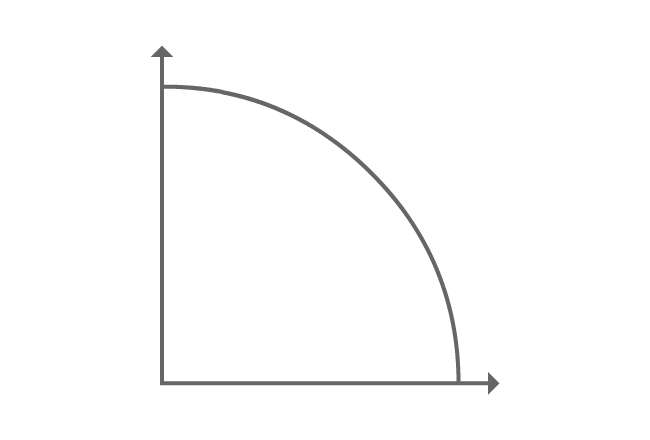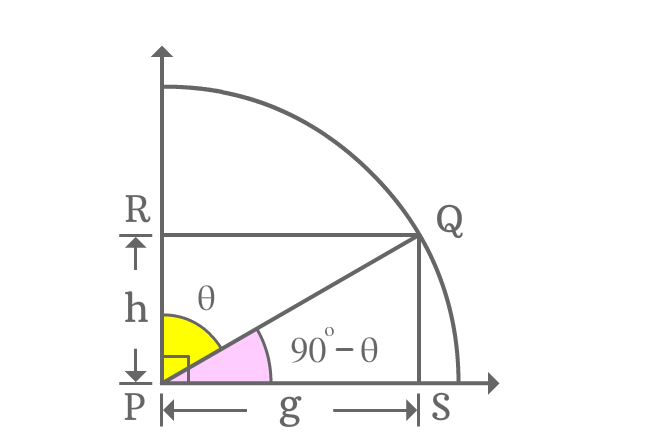# Cofunction identity of tan function

## Formula

Sexagesimal System
$\tan{(90^\circ-\theta)} \,=\, \cot{\theta}$
$\tan{\Big(\dfrac{\pi}{2}-x\Big)} \,=\, \cot{x}$

In this trigonometric rule, theta is used to represent angle in degrees and x is used to represent angle in radians.

Tan function contains an allied angle which belongs to first quadrant. So, the formula is called as first quadrant’s allied angle identity of tan function. Similarly, the angles of both tan and cot functions are complementary angles. Therefore, it is usually called as cofunction identity of tan function.

### ProofIn first quadrant, a line segment is rotated to an angle and the line segment is called as $\overline{PQ}$.

Perpendicular lines are drawn to horizontal and vertical lines from point $Q$ and they intersect perpendicular rays at points $S$ and $R$ respectively. Thus, it constructed two right angled triangles geometrically, known as $\Delta QPR$ and $\Delta QPS$.

Assume $\angle QPR = \theta$, then $\angle QPS = 90^\circ-\theta$.

Assume $PS = g$, then $RQ = g$ and if $RP = h$, then $QS = h$ because of equality and parallelism of the line segments.

#### Express tan of allied angleThe angle of $\Delta QPS$ is an allied angle and it is $90^\circ-\theta$. Express tan of first quadrant’s allied angle in mathematical form by expressing it in the form of ratio of the associated sides.

$\tan{(90^\circ-\theta)} = \dfrac{QS}{PS}$

The lengths of both opposite and adjacent sides are known, and they are $h$ and $g$ respectively.

$\implies \tan{(90^\circ-\theta)} = \dfrac{h}{g}$

#### Find equivalent value of the fractionTan of allied angle of first quadrant is expressed as a fraction of the lengths of the sides and express the same fraction as an equivalent trigonometric function. It is possible by the $\Delta QPR$.

$\dfrac{h}{g} = \dfrac{PR}{QR}$

As per $\Delta QPR$, the angle of the triangle is theta. The ratio of lengths of $\overline{PR}$ to $\overline{QR}$ is cot of angle theta.

$\implies \dfrac{h}{g} = \cot{\theta}$

#### Cofunction identity of tan function

The previous two steps have proved geometrically that

$\tan{(90^\circ-\theta)}$ $=$ $\dfrac{h}{g}$ $=$ $\cot{\theta}$

$\therefore \,\,\,\,\,\, \tan{(90^\circ-\theta)} \,=\, \cot{\theta}$

Geometrically, it is proved that tan of first quadrant’s allied angle is equal to cot of angle. Hence, this trigonometric identity is known as first quadrant’s allied angle identity of tan function. But, the angles of both tan and cot functions are complementary angles. Due to this reason, this rule is known as cofunction identity of tan function.

Latest Math Topics
Jun 26, 2023
Jun 23, 2023

###### Math Questions

The math problems with solutions to learn how to solve a problem.

Learn solutions

Practice now

###### Math Videos

The math videos tutorials with visual graphics to learn every concept.

Watch now

###### Subscribe us

Get the latest math updates from the Math Doubts by subscribing us.